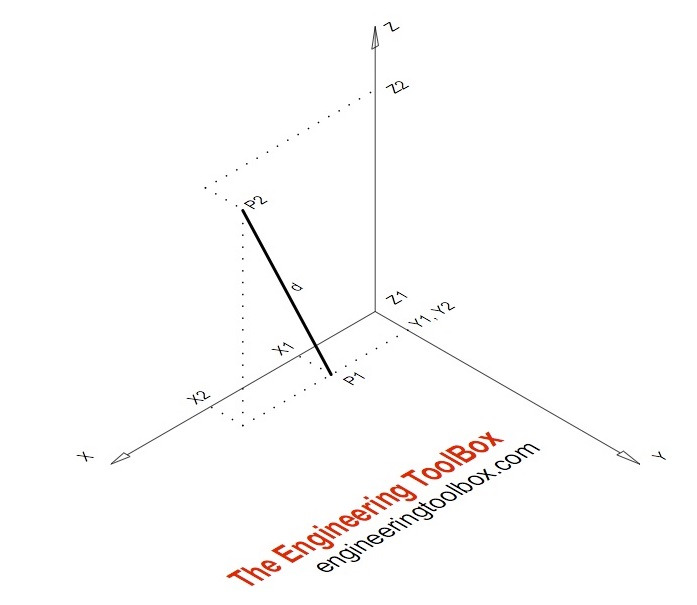Engineering ToolBox - Resources, Tools and Basic Information for Engineering and Design of Technical Applications!

# Distance between 3D Points

## Distance between two points in a three dimension x, y and z coordinate system - online calculator.

The distance between two points in a three dimensional - 3D - coordinate system can be calculated as

d = ((x2 - x1)2 + (y2 - y1)2 + (z2 - z1)2)1/2                                 (1)

where

d = distance (m, inches ...)

x, y, z = coordinates

### Example - the Distance between two points in a three dimensional spaceThe distance between point P1(1,1,0) and point P2(2,1,2)  can be calculated as

d = ((2 - 1)2 + (1 - 1)2 + (2 - 0)2)1/2

= 2.24

xyz
P1
P2

## Related Topics

• ### Mathematics

Mathematical rules and laws - numbers, areas, volumes, exponents, trigonometric functions and more.

## Related Documents

• ### Acceleration of Gravity vs. Latitude and Elevation

Acceleration of gravity due to latitude and elevation above sea level.
• ### Area Survey App

Online app that can be used to make an exact plot of a surveyed area - like a room, a property or any 2D shape.
• ### Cartesian Coordinate System - Distance and Intermediate Position Between Two Points

Distance and intermediate position between two point in a cartesian x and y coordinate system.
• ### Circle - Equation

The equation for a circle
• ### Latitude and Longitude Converter

Convert between Degrees, Minutes and Seconds and Decimal Units (or vice versa).
• ### Polar vs. Cartesian Coordinates

Convert between Cartesian and Polar coordinates.
• ### UTM to Latitude and Longitude Converter

Free online UTM to Latitude and Longitude coordinates converter.

## Engineering ToolBox - SketchUp Extension - Online 3D modeling!

Add standard and customized parametric components - like flange beams, lumbers, piping, stairs and more - to your Sketchup model with the Engineering ToolBox - SketchUp Extension - enabled for use with older versions of the amazing SketchUp Make and the newer "up to date" SketchUp Pro . Add the Engineering ToolBox extension to your SketchUp Make/Pro from the Extension Warehouse !

We don't collect information from our users. More about

## Citation

• The Engineering ToolBox (2013). Distance between 3D Points . [online] Available at: https://www.engineeringtoolbox.com/distance-relationship-between-two-points-d_1854.html [Accessed Day Month Year].

Modify the access date according your visit.

9.19.12